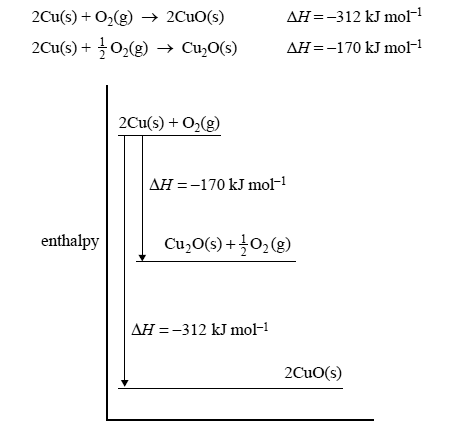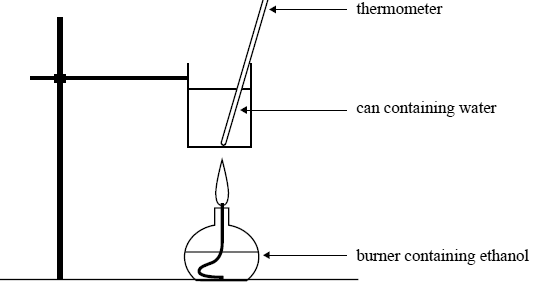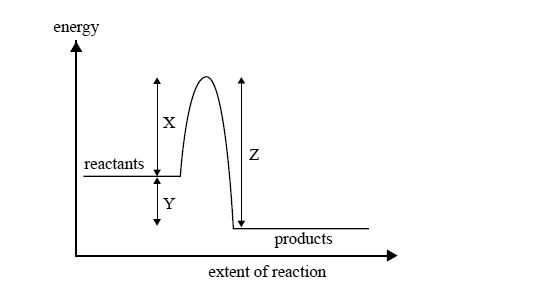Thermochemistry 2008 VCE The following energy profile relates to the two reactionsThe value of ΔH, in kJ mol–1, for the reaction 4CuO(s) → 2Cu2O(s) + O2(g) is A. +284 B. +142 C. –142 D. –284 Solution A foam cup calorimeter containing 100 mL of water is calibrated by passing an electric current through a small heater placed in the solution. Assuming that all measurements are accurate, which one of the following is the most likely calibration factor (in J°C–1) for the calorimeter and contents? A. 120 B. 240 C. 480 D. 960 Solution The numerical value of the heat of combustion of 1-propanol in kJ g–1 is A. 33.60 B. 2016 C. 3.360 × 104 D. 1.210 × 105 SolutionTwo experiments are carried out. Both involve the combustion of 2.09g of ethanol. Experiment 1 Ethanol is used to calibrate a bomb calorimeter. 2.09 g of ethanol is placed in the bomb calorimeter and reacted with excess oxygen. After the reaction is complete, the temperature of the water surrounding the bomb in the calorimeter has increased by 33.2°C. Calculate, to an appropriate number of signifi cant figures, the calibration factor of the calorimeter, in kJ°C–1. Solution The same mass of ethanol is burnt to heat 200 g of water in a can as shown in the following diagram.Initial temperature of water in the can: 25.3°C Mass of water in the can: 200 g Mass of ethanol burnt: 2.09 g Calculate the final temperature of the water in the can. Assume that 60% of the heat from the burning ethanol is transferred to the water. SolutionSince the start of the industrial age, most of the energy used by humans has come from the burning of coal and oil. In that time the amount of CO2 in the air has increased from approximately 0.42% by mass to 0.58% by mass. Assume that the total mass of the earth’s atmosphere is 5.15 × 1018 kg. Calculate the additional mass of CO2, in kg, that has been added to the earth’s atmosphere since the start of the industrial age. Solution If half of this additional CO2 has come from the burning of coal, calculate the total amount of energy, in kJ, that has been produced by burning all this coal, given that C(s) + O2(g) → CO2(g); ΔH = –394 kJ mol–1 For the purposes of this calculation, assume that coal is pure carbon. SolutionThe following reaction can occur to completion in aqueous solution. CH3Cl(aq) + OH–(aq) → CH3OH(aq) + Cl–(aq) The energy change during this process is illustrated byA reaction can occur between a CH3Cl molecule and a hydroxide ion A. every time they collide. B. only when they collide with exactly the energy X. C. only when they collide with an energy equal to Y–Z. D. only when they collide with an energy greater than or equal to energy X. Solution A catalyst appropriate for this reaction will affect the value of A. X only. B. Y only. C. X and Z only. D. X, Y and Z. Solution